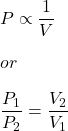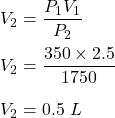## A gas occupies a volume of 2.50 L at a pressure of 350.0 kPa. If the temperature remains constant, what volume would the gas occupy at

Question

A gas occupies a volume of 2.50 L at a pressure of 350.0 kPa. If the temperature remains
constant, what volume would the gas occupy at 1750 kPa ?
1. 12.5 L
2. 5.00 L
3. 1.40e+2L
4. 0.500 L

in progress 0
2 months 2021-07-28T16:59:56+00:00 1 Answers 4 views 0

V₂ = 0.5 L

Explanation:

Given that,

Initial volume, V₁ = 2.5 L

Pressure, P₁ = 350 kPa

New pressure, P₂ = 1750 kPa

We need to find the new volume if the temperature remains constant. The mathematical relation between P and V is :Where

V₂ is new volume

So,So, the new volume is equal to 0.5 L.